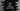# How to pass an array to function in Java## Example to pass an array to a function in Java:

In this post, we will learn how to pass an Array to a function or method in Java. We can pass an array to a function in a similar way we do it for other arguments.

Let’s learn how the array is passed and how to access the items in this array.

### Passing an array to a function in Java:

We can pass an array to a function and this is passed as reference, i.e. if we make any changes to the array inside the function, it will reflect in the caller function.

For example:

``````import java.util.Arrays;

class Main {
private static void modifyArray(int[] arr) {
arr = -1;
arr = -1;
}

public static void main(String[] args) {
int[] givenArray = {1, 2, 3, 4, 5};

System.out.println("Given array: " + Arrays.toString(givenArray));

modifyArray(givenArray);

System.out.println("Given after modifyArray is called: " + Arrays.toString(givenArray));
}
}``````

In this example,

• givenArray is an array of integers.
• modifyArray is a method that takes an array as the parameter and changes the items at index 1 and 2 to -1.
• We are printing the array content before and after modifyArray is called.

If you run this program, it will print the below output:

``````Given array: [1, 2, 3, 4, 5]
Given after modifyArray is called: [1, -1, -1, 4, 5]``````

So, you can see here that the original array content is changed if we make any change in the method. This is because the array is passed as a reference.

### Create a local variable in the function:

Suppose you create a local variable in the function and assign the parameter array to this variable:

``````import java.util.Arrays;

class Main {
private static void modifyArray(int[] arr) {
int[] arr2 = arr;
arr2 = -1;
arr2 = -1;
}

public static void main(String[] args) {
int[] givenArray = {1, 2, 3, 4, 5};

System.out.println("Given array: " + Arrays.toString(givenArray));

modifyArray(givenArray);

System.out.println("Given after modifyArray is called: " + Arrays.toString(givenArray));
}
}``````

It will actually print the same result. Because we are not copying the data of arr to arr2. Both arr2 and arr are referring to the same array or memory block.

If you run this, it will print:

``````Given array: [1, 2, 3, 4, 5]
Given after modifyArray is called: [1, -1, -1, 4, 5]``````

### Passing more than one array to a function:

We can also pass more than one array to a function. We need to define these arrays as parameters for a method and we can call this method from anywhere we want. For example, let’s try to find the size of the larger array by using a different function:

``````class Main {
private static int findMaxLength(int[] arr1, int[] arr2) {
return Math.max(arr1.length, arr2.length);
}

public static void main(String[] args) {
int[] firstArray = {1, 2, 3, 4, 5};
int[] secondArray = {1, 2, 3, 4};

System.out.println("Length of the larger array is: " + findMaxLength(firstArray, secondArray));
}
}``````

Here,

• We are passing two arrays to the function findMaxLength.
• It uses Math.max to find the larger array length and returns that length.
• This length value is printed in the main method.

If you run this program, it will print 5 as this is the length of the larger array.

``Length of the larger array is: 5``

Passing an array as a parameter is similar to passing any other variables as the parameters to a function. The difference is that it is passed as a reference.